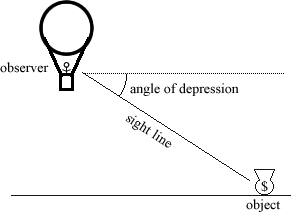# angle of depression

Angle of Depression

The angle below horizontal that an observer must look to see an object that is lower than the observer. Note: The angle of depression is congruent to the angle of elevation (this assumes the object is close enough to the observer so that the horizontals for the observer and the object are effectively parallel; this would not be the case for an astronaut in orbit around the earth observing an object on the ground).